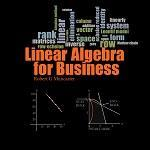My AccountMATH 125: Elementary Linear Algebra eText

\$16.00
Electronic Textbook
Eligibility: UIUC Faculty and Staff, UIUC Students and WebStore Administrators.
MATH 125: Elementary Linear Algebra eText
WEBASSIGN CODES SOLD THROUGH SEPARATE OFFER (SEE BELOW)
Total Price:\$16.00
Payment Type: Credit Card

Description

Select this product to purchase an activation code for the eText https://etext.illinois.edu/  MATH 125

WEBASSIGN CODES SOLD THROUGH SEPARATE OFFER

Please note that eTexts are non-refundable even if you drop the course.

Chapter 1: Linear Programming in the Plane

• Section 1: Linear inequalities in the plane
• Section 2: Solving linear programs in the plane
• Section 3: Applications of linear programming

Chapter 2: Matrices and Linear Systems

• Section 1: Row operations and Gaussian elimination
• Section 2: Reduced row echelon form
• Section 3: Consistency and row rank
• Section 4: Euclidean 3-space
• Section 5: Linear programming and the simplex algorithm
• Section 6: Simplex algorithm - additional considerations

Chapter 3: Introduction to Vectors

• Section 1: Vectors and lines in 2D and nD
• Section 2: Dot products and orthogonality
• Section 3: Linear combinations

Chapter 4: Matrices and Applications

• Section 1: Matrix operations
• Section 2: The inverse of a matrix
• Section 3: The Leontief open model
• Section 4: Markov analysis

Chapter 5: Production Schedule Analysis (Vector Spaces)

• Section 1: Three fundamental examples
• Section 2: Spanning sets and geometry
• Section 3: Subspaces
• Section 4: Linear dependence and independence
• Section 5: Bases and basis selection
• Section 6: Approximations (projections)
• Section 7: Approximations (least squares)